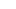MACD is an acronym for Moving Average Convergence Divergence.

This technical indicator is a tool that’s used to identify moving averages that are indicating a new trend, whether it’s bullish or bearish.

After all, a top priority in trading is being able to find a trend, because that is where the most money is made.With a MACD chart, you will usually see three numbers that are used for its settings.

• The first is the number of periods that are used to calculate the faster-moving average.
• The second is the number of periods that are used in the slower-moving average.
• And the third is the number of bars that are used to calculate the moving average of the difference between the faster and slower moving averages.

For example, if you were to see “12, 26, 9” as the MACD parameters (which is usually the default setting for most charting software), this is how you would interpret it:

• The 12 represents a moving average of the previous 12 bars.
• The 26 represents a moving average of the previous 26 bars.
• The 9 represents a moving average of the difference between the two moving averages above.

## Misconceptions

There is a common misconception when it comes to the lines of the MACD.

There are two lines:

1. The “MACD Line
2. The “Signal Line

The two lines that are drawn are NOT moving averages of the price.

The MACD Line is the difference (or distance) between two moving averages. These two moving averages are usually exponential moving averages (EMAs).

When looking at the indicator, they consider the MACD Line the “faster” moving average.

In our example above, the MACD Line is the difference between the 12 and 26-period moving averages.